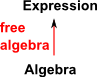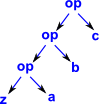# Maths - Expressions

Here are some concepts which are used in functional programming (see: Meijer, Erik; Fokkinga, Maarten; Paterson, Ross. "Functional Programming with Bananas, Lenses, Envelopes, and Barbed Wire") and these concepts are also used in category theory.

Notation Functional Programming Term Category Theory Term Meaning (from here)
banana catamorphism tears down a structure level by level
[( )] lense anamorphism builds up a structure level by level
envelope hylomorpism builds up and tears down a virtual structure
barbed wire paramorphism tears down a structure with primitive recursion

### Catamorphism and Anamorphism

 If we start with an algebra that has a binary operation (S,S)->S and, instead of supplying interal values we supply a symbolic value to represent a variable or unknown, we might build up a free algebra.• Catamorphism - initial algebra -> other algebra.
• Anamorphism

## Catamorphism (banana)

Reduces the data structure to a required value.

#### Functional Programming Examples:

• Generalise the concept of fold.

#### Category:

Tree is a initial object in the category of F-algebras

## Anamorphism (Lenses)

Upwards morphism - Builds an invocation tree as an explicit data structure.

#### Functional Programming Examples:

• Generalise the concept of unfold
• zip and iterate are examples of anamorphisms

A notation for ana(f) found in the literature is [(f)]. The brackets used are known as lens brackets.

In programming 'lenses' can be used as the functional equivalent of a 'reference' and they allow a functional (non-mutable) data structure to be used like an imperative (mutable) data structure. They do this by duplicating the data rather than using references to the same stucture. This allows functional programs to act on imperitive structures like databases in a purely functional way. Unlike other ways of doing this lenses are composable, that is, we can nest these structures to any debth (subject to memory constraints because of this duplication).

#### Laws

 get-put P(S,G (S))=S put-get G(P(S,V))=V

Where:

• P( , ) = put
• G( ) = get

```case class Lense[A,B] {
g: A => B
s: (B,A) => A
| {
DEF get (a:A):B=g(a)
DEF set(b:B,a:A): A=s(b,a)
```

## Hylomorphism

Composition of an anamorphism and a catamorphism. Invocation tree is isomorphic to a function which processes lists.

### Example Monoid

free monoid = list

• Fold is a catamorphism
• Unfold is a anamorphismFold LeftFold RightSo the fold operation takes a list and a monoid and returns a value### Example Field

free field = tree?Where I can, I have put links to Amazon for books that are relevant to the subject, click on the appropriate country flag to get more details of the book or to buy it from them.The Princeton Companion to Mathematics - This is a big book that attempts to give a wide overview of the whole of mathematics, inevitably there are many things missing, but it gives a good insight into the history, concepts, branches, theorems and wider perspective of mathematics. It is well written and, if you are interested in maths, this is the type of book where you can open a page at random and find something interesting to read. To some extent it can be used as a reference book, although it doesn't have tables of formula for trig functions and so on, but where it is most useful is when you want to read about various topics to find out which topics are interesting and relevant to you.